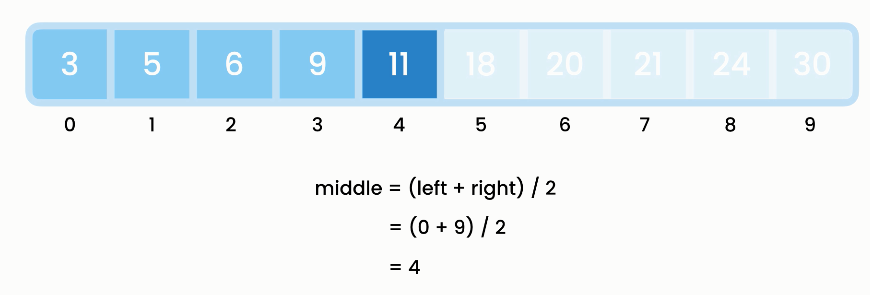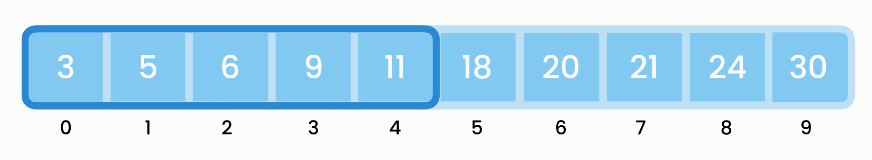Search Algorithms

# Search Algorithms

2020-01-14T23:46:37.233Z

## Common search algorithms

Item-by-item search for an item in an array. Best-case scenario runtime complexity is `O(1)`; for worst-case scenario, it is `O(n)`.

``````public int linearSearch(int[] array, int target) {
for (var i = 0; i < array.length; i++)
if (array[i] == target)
return i;
return -1;
}
``````

Only for sorted lists. First, sort; then, look at the middle item using the formula: `middle = (left + right) / 2`, dividing and searching recursively.Binary search
Best
Time complexity `O(log n)`
Space complexity with recursion `O(log n)`
Space complexity with iteration `O(1)`

For every time we double the number of items in the ordered array, the number of steps needed for binary search increases by just one. This pattern is unusually efficient: for every time we double the data, the binary search algorithm adds a maximum of just one more step. For linear search, every time we double the number of elements in the array, we double the number of steps we need to find something. For binary search, every time we double the number of elements in the array, we only need to add one more step.

Implementing binary search with recursion:

``````public int binarySearchRecursive(int[] array, int target) {
return binarySearchRecursive(array, target, 0, array.length - 1);
}

private int binarySearchRecursive(int[] array, int target, int left, int right) {

// base condition
if (right < left)
return -1;

int middle = (left + right) / 2;
if (array[middle] == target)
return middle;
if (target < array[middle])
return binarySearchRecursive(array, target, left, middle - 1);

return binarySearchRecursive(array, target, middle + 1, right);
}
``````

Implementing binary search with iteration:

``````public int binarySearchIterative(int[] array, int target) {
var left = 0;
var right = array.length - 1;

while (left <= right) {
var middle = (left + right) / 2;
if (array[middle] == target)
return middle;
if (target < array[middle])
right = middle - 1;
else
left = middle + 1;
}

return -1;
}
``````

## Unusual search algorithms

Instead of dividing the list into halves at every step, we divide it into three parts with `mid1` and `mid2`. Best-case scenario runtime complexity is `O(log base 3 n)`.

Binary search is faster than ternary search.

Use the formula:

• partitionSize = (right - left) / 3
• mid1 = left + partitionSize
• mid2 = right - partitionSize
``````public int ternarySearch(int[] array, int target, int left, int right) {
int partitionSize = (right - left) / 3;
int mid1 = left + partitionSize;
int mid2 = right - partitionSize;

// base condition
if (left > right)
return -1;

if (array[mid1] == target)
return mid1;

if (array[mid2] == target)
return mid2;

// if target is in left partition, which goes
// from left to mid - 1
if (target < array[mid1])
return ternarySearch(array, target, left, mid - 1);

// if target is in right partition, which goes
// from mid + 1 to right
if (target > array[mid2])
return ternarySearch(array, target, mid2 + 1, right);

// if not in left and right partition, target is in
// middle partition
return ternarySearch(array, target, mid1 + 1, mid2 - 1);
}
``````

Improvement to linear search. Divide list into blocks and jump to block where target may exist. Then do linear search only in that block.

The ideal number of blocks is the square root of numbers in the array.

``````public int jumpSearch(int[] array, int target) {
int blockSize = (int) Match.sqrt(array.length);
int start = 0;
int next = blockSize; // i.e. start of next block

while (start < array.length &&
array[next - 1] < target) {
start = next;

next += blockSize;
if (next > array.length)
next = array.length;
}
for (var i = start; i < next; i++)
if (array[i] == target)
return i;

return -1;
}
``````

Start with a small range, see it item is in small range. If not double the range and search again at each step.Exponential search
``````public int exponentialSearch(int[] array, int target) {
int bound = 1;
while (bound < array.length &&
array[bound] < target)
bound *= 2;

int left = bound / 2;
int right = Math.min(bound, array.length - 1);
return binarySearchRecursive(array, target, left, right);
}
``````